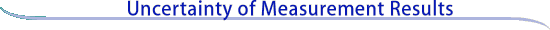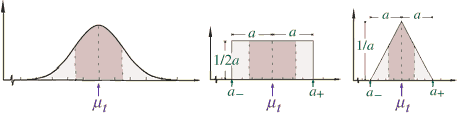Evaluating uncertainty components: Type B

A Type B evaluation of standard uncertainty is usually based on scientific judgment using all of the relevant information available, which may include:

• previous measurement data,
• experience with, or general knowledge of, the behavior and property of relevant materials and instruments,
• manufacturer's specifications,
• data provided in calibration and other reports, and
• uncertainties assigned to reference data taken from handbooks.

Below are some examples of Type B evaluations in different situations, depending on the available information and the assumptions of the experimenter. Broadly speaking, the uncertainty is either obtained from an outside source, or obtained from an assumed distribution.

 Uncertainty obtained from an outside source

Multiple of a standard deviation

Procedure:  Convert an uncertainty quoted in a handbook, manufacturer's specification, calibration certificate, etc., that is a stated multiple of an estimated standard deviation to a standard uncertainty by dividing the quoted uncertainty by the multiplier.

Confidence interval

Procedure:  Convert an uncertainty quoted in a handbook, manufacturer's specification, calibration certificate, etc., that defines a "confidence interval" having a stated level of confidence, such as 95 % or 99 %, to a standard uncertainty by treating the quoted uncertainty as if a normal probability distribution had been used to calculate it (unless otherwise indicated) and dividing it by the appropriate factor for such a distribution. These factors are 1.960 and 2.576 for the two levels of confidence given.Uncertainty obtained from an assumed distribution

Normal distribution: "1 out of 2"

Procedure:  Model the input quantity in question by a normal probability distribution and estimate lower and upper limits a- and a+ such that the best estimated value of the input quantity is (a+ + a-)/2 (i.e., the center of the limits) and there is 1 chance out of 2 (i.e., a 50 % probability) that the value of the quantity lies in the interval a- to a+. Then uj is approximately 1.48 a, where a = (a+ - a-)/2 is the half-width of the interval.

Normal distribution: "2 out of 3"

Procedure:  Model the input quantity in question by a normal probability distribution and estimate lower and upper limits a- and a+ such that the best estimated value of the input quantity is (a+ + a-)/2 (i.e., the center of the limits) and there are 2 chances out of 3 (i.e., a 67 % probability) that the value of the quantity lies in the interval a- to a+. Then uj is approximately a, where a = (a+ - a-)/2 is the half-width of the interval.

Normal distribution: "99.73 %"

Procedure:  If the quantity in question is modeled by a normal probability distribution, there are no finite limits that will contain 100 % of its possible values. However, plus and minus 3 standard deviations about the mean of a normal distribution corresponds to 99.73 % limits. Thus, if the limits a- and a+ of a normally distributed quantity with mean (a+ + a-)/2 are considered to contain "almost all" of the possible values of the quantity, that is, approximately 99.73 % of them, then uj is approximately a/3, where a = (a+ - a-)/2 is the half-width of the interval.

Uniform (rectangular) distribution

Procedure:  Estimate lower and upper limits a- and a+ for the value of the input quantity in question such that the probability that the value lies in the interval a- and a+ is, for all practical purposes, 100 %. Provided that there is no contradictory information, treat the quantity as if it is equally probable for its value to lie anywhere within the interval a- to a+; that is, model it by a uniform (i.e., rectangular) probability distribution. The best estimate of the value of the quantity is then (a+ + a-)/2 with uj = a divided by the square root of 3, where a = (a+ - a-)/2 is the half-width of the interval.

Triangular distribution

The rectangular distribution is a reasonable default model in the absence of any other information. But if it is known that values of the quantity in question near the center of the limits are more likely than values close to the limits, a normal distribution or, for simplicity, a triangular distribution, may be a better model.

Procedure:  Estimate lower and upper limits a- and a+ for the value of the input quantity in question such that the probability that the value lies in the interval a- to a+ is, for all practical purposes, 100 %. Provided that there is no contradictory information, model the quantity by a triangular probability distribution. The best estimate of the value of the quantity is then (a+ + a-)/2 with uj = a divided by the square root of 6, where a = (a+ - a-)/2 is the half-width of the interval.

Schematic illustration of probability distributions
The following figure schematically illustrates the three distributions described above: normal, rectangular, and triangular. In the figures, µt is the expectation or mean of the distribution, and the shaded areas represent ± one standard uncertainty u about the mean. For a normal distribution, ± u encompases about 68 % of the distribution; for a uniform distribution, ± u encompasses about 58 % of the distribution; and for a triangular distribution, ± u encompasses about 65 % of the distribution.Continue to Combining uncertainty components